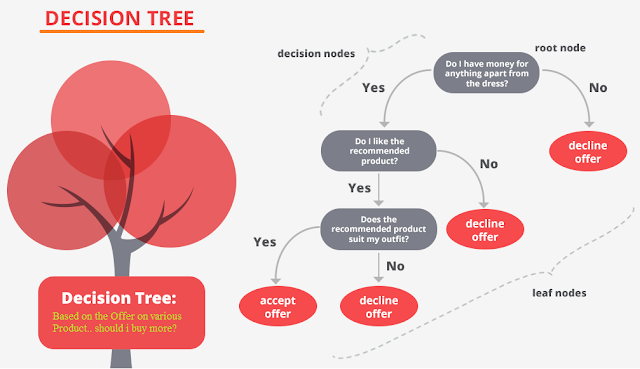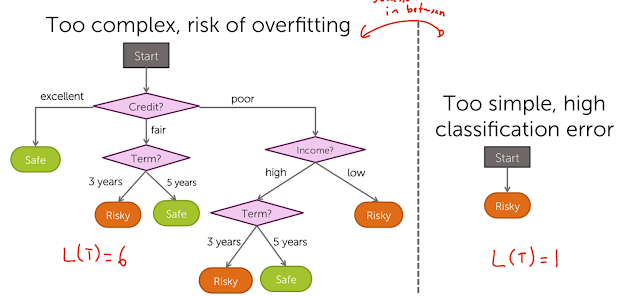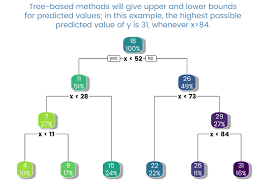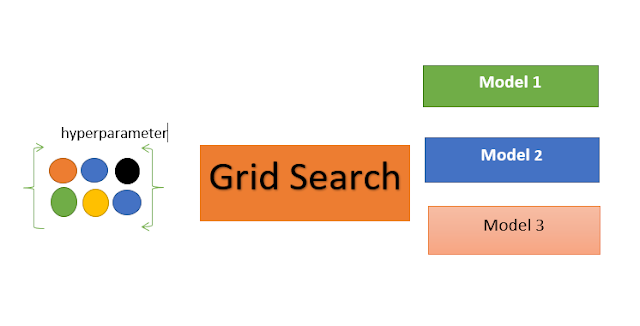Latest News

## Friday, September 1, 2023

The level of interdependence between the variables in your dataset should be identified and quantified. By being aware of these interdependencies, you can better prepare your data to match the demands of machine learning algorithms like linear regression, whose performance will suffer as a result.

You will learn how to compute correlation for various types of variables and relationships in this course. Correlation is the statistical summary of the relationship between variables.

There are 4 sections in this tutorial; they are as follows:

1. Correlation: What Is It?
2. Covariance of the Test Dataset
3. Correlation by Pearson
4. Using Spearman's Correlation

Correlation: What Is It?

There are many different ways that variables in a dataset might be related or connected.
For instance:
• The values of one variable may influence or be dependent upon the values of another.
• There may be a very slight correlation between two variables.
• A third unknowable variable may be dependent on two other variables.
The ability to better grasp the relationships between variables can be helpful in data analysis and modeling. The term "correlation" refers to the statistical association between two variables.

A correlation can be either positive or negative, meaning that when one variable's value changes, the other variable's value also changes in the same way. The variables may not be correlated if the correlation is 0 or neutral.
1. Both variables fluctuate in the same direction when there is a positive correlation.
2. No correlation exists between the changes in the variables in a neutral correlation.
3. In a negative correlation, the variables shift against each other.

### Impact of Correlations on ML Models:

• Multicollinearity, or the close relationship between two or more variables, might cause some algorithms to perform worse. For instance, in linear regression, one of the problematic associated variables should be eliminated to raise the model's accuracy.
• In order to gain insight into which variables may or may not be relevant as input for constructing a model, we may also be interested in the correlation between input variables and the output variable.

### Generating Data for the Correlation Analysis:

# generate related variables
from numpy import mean
from numpy import std
from numpy.random import randn
from numpy.random import seed
from matplotlib import pyplot
# seed random number generator
seed(1)
# prepare data
data1 = 20 * randn(1000) + 100
data2 = data1 + (10 * randn(1000) + 50)
# summarize
print('data1: mean=%.3f stdv=%.3f' % (mean(data1), std(data1)))
print('data2: mean=%.3f stdv=%.3f' % (mean(data2), std(data2)))
# plot
pyplot.scatter(data1, data2)
pyplot.show()

data1: mean=100.776 stdv=19.620 data2: mean=151.050 stdv=22.358
Covariance pertains to the potential association between variables through a linear connection, where this connection remains consistently additive across both sets of data.

This connection can be succinctly described as the covariance between two variables. It is determined by averaging the product of values from each data set, after those values have been adjusted to be centered (by subtracting their mean).

#### The formula for computing the sample covariance is outlined as follows:

cov(X, Y) = (sum (x - mean(X)) * (y - mean(Y)) ) * 1/(n-1)

Utilizing the mean in the computation implies a requirement for Gaussian or Gaussian-like distribution in each data sample. Covariance's sign signifies whether variables change together (positive) or diverge (negative). Magnitude's interpretation is complex. A covariance of zero means full independence.

NumPy's cov() function computes a covariance matrix for multiple variables.
covariance = cov(data1, data2)

The matrix's diagonal holds the self-covariance of each variable. The other entries signify the covariance between the paired variables; given that only two variables are under consideration, these remaining entries are identical.

We can derive the covariance matrix for the given pair of variables in our test scenario. Here's the complete illustration:

from numpy.random import randn
from numpy.random import seed
from numpy import cov

# Set random seed
seed(1)

# Prepare data
data1 = 20 * randn(1000) + 100
data2 = data1 + (10 * randn(1000) + 50)

# Calculate covariance matrix
covariance = cov(data1, data2)
print(covariance)

[[385.33297729 389.7545618 ] [389.7545618 500.38006058]]

Covariance and covariance matrix play a crucial role in statistics and multivariate analysis for describing relationships among variables.

By executing the example, the covariance matrix is computed and displayed.

Since the dataset involves variables drawn from Gaussian distribution and exhibits linear correlation, covariance is a suitable approach for characterization.

The covariance between the two variables measures 389.75. This positive value indicates that the variables change in the expected direction together.

Using covariance as a standalone statistical tool is problematic due to its complex interpretation, prompting the introduction of Pearson's correlation coefficient.

### Pearson’s Correlation:

The Pearson correlation coefficient, named after Karl Pearson, summarizes linear relationship strength between data samples.

It's calculated by dividing the covariance of variables by the product of their standard deviations, normalizing the covariance for an interpretable score.

Pearson's correlation coefficient = covariance(X, Y) / (stdv(X) * stdv(Y))

The requirement for mean and standard deviation use implies a Gaussian or Gaussian-like distribution for the data samples.

The outcome of the calculation, the correlation coefficient, provides insights into the relationship.

The coefficient ranges from -1 to 1, signifying the extent of correlation. 0 implies no correlation. Typically, values below -0.5 or above 0.5 indicate significant correlation, while values below these thresholds suggest weaker correlation.

To compute the Pearson’s correlation coefficient for data samples of equal length, one can utilize the pearsonr() function from SciPy.

# calculate the Pearson's correlation between two variables
from numpy.random import randn
from numpy.random import seed
from scipy.stats import pearsonr
# seed random number generator
seed(1)
# prepare data
data1 = 20 * randn(1000) + 100
data2 = data1 + (10 * randn(1000) + 50)
# calculate Pearson's correlation
corr, _ = pearsonr(data1, data2)
print('Pearsons correlation: %.3f' % corr)

Pearsons correlation: 0.888

The variables show a strong positive correlation with a coefficient of 0.8, indicating high association, similar to values near 1.0.

Pearson's correlation coefficient assesses relationships among multiple variables. This involves creating a correlation matrix by calculating interactions between each variable pair. The matrix is symmetrical, with 1.0 on the diagonal due to perfect self-correlation in each column.

### Spearman’s Correlation

Variables can exhibit varying nonlinear relationships across their distributions. The distribution of these variables may also deviate from the Gaussian pattern.

For such cases, the Spearman's correlation coefficient, named after Charles Spearman, measures the strength of association between data samples. It's applicable for both nonlinear and linear relationships, with slightly reduced sensitivity (lower coefficients) in the latter case.

Similar to the Pearson correlation, scores range between -1 and 1 for perfect negative and positive correlations, respectively. However, Spearman's coefficient utilizes rank-based statistics rather than sample values, making it suitable for non-parametric analysis, where data distribution assumptions like Gaussian aren't made.

Spearman's correlation coefficient
= covariance(rank(X), rank(Y)) / (stdv(rank(X)) * stdv(rank(Y)))
# calculate the spearmans's correlation between two variables
from numpy.random import randn
from numpy.random import seed
from scipy.stats import spearmanr
# seed random number generator
seed(1)
# prepare data
data1 = 20 * randn(1000) + 100
data2 = data1 + (10 * randn(1000) + 50)
# calculate spearman's correlation
corr, _ = spearmanr(data1, data2)
print('Spearmans correlation: %.3f' % corr)

```Spearmans correlation: 0.872
```

Despite assuming Gaussian data and a linear variable relationship, the nonparametric rank-based method reveals a robust 0.8 correlation between the variables.

## Wednesday, August 30, 2023

Question:

Lanternfish

You are in presence of specific species of lanternfish. They have one special attribute, each lanternfish creates a new lanternfish once every 7 days.

However, this process isn’t necessarily synchronized between every lanternfish - one lanternfish might have 2 days left until it creates another lanternfish, while another might have 4. So, you can model each fish as a single number that represents the number of days until it creates a new lanternfish.

Furthermore, you reason, a new lanternfish would surely need slightly longer before it’s capable of producing more lanternfish: two more days for its first cycle.

So, suppose you have a lanternfish with an internal timer value of 3:

After one day, its internal timer would become 2.

After another day, its internal timer would become 1.

After another day, its internal timer would become 0.

After another day, its internal timer would reset to 6, and it would create a new lanternfish with an internal timer of 8.

After another day, the first lanternfish would have an internal timer of 5, and the second lanternfish would have an internal timer of 7.

A lanternfish that creates a new fish resets its timer to 6, not 7 (because 0 is included as a valid timer value). The new lanternfish starts with an internal timer of 8 and does not start counting down until the next day.

For example, suppose you were given the following list:

3,4,3,1,2

This list means that the first fish has an internal timer of 3, the second fish has an internal timer of 4, and so on until the fifth fish, which has an internal timer of 2. Simulating these fish over several days would proceed as follows:

Initial state: 3,4,3,1,2

After 1 day: 2,3,2,0,1

After 2 days: 1,2,1,6,0,8

After 3 days: 0,1,0,5,6,7,8

After 4 days: 6,0,6,4,5,6,7,8,8

After 5 days: 5,6,5,3,4,5,6,7,7,8

After 6 days: 4,5,4,2,3,4,5,6,6,7

After 7 days: 3,4,3,1,2,3,4,5,5,6

After 8 days: 2,3,2,0,1,2,3,4,4,5

After 9 days: 1,2,1,6,0,1,2,3,3,4,8

After 10 days: 0,1,0,5,6,0,1,2,2,3,7,8

After 11 days: 6,0,6,4,5,6,0,1,1,2,6,7,8,8,8

After 12 days: 5,6,5,3,4,5,6,0,0,1,5,6,7,7,7,8,8

After 13 days: 4,5,4,2,3,4,5,6,6,0,4,5,6,6,6,7,7,8,8

After 14 days: 3,4,3,1,2,3,4,5,5,6,3,4,5,5,5,6,6,7,7,8

After 15 days: 2,3,2,0,1,2,3,4,4,5,2,3,4,4,4,5,5,6,6,7

After 16 days: 1,2,1,6,0,1,2,3,3,4,1,2,3,3,3,4,4,5,5,6,8

After 17 days: 0,1,0,5,6,0,1,2,2,3,0,1,2,2,2,3,3,4,4,5,7,8

After 18 days: 6,0,6,4,5,6,0,1,1,2,6,0,1,1,1,2,2,3,3,4,6,7,8,8,8,8

Each day, a 0 becomes a 6 and adds a new 8 to the end of the list, while each other number decreases by 1 if it was present at the start of the day.

In this example, after 18 days, there are a total of 26 fish.

Question 1 (easy): How many lanternfish would there be after 80 days?

Question 2 (harder): How many lanternfish would there be after 400 days?

## Wednesday, July 26, 2023

What is Llama2?

Llama 2, the next generation of our open source large language model.

• LLama2 is a transformer-based language model developed by researchers at Meta AI.
• The model is trained on a large corpus of text data and is designed to generate coherent and contextually relevant text.
•  LLama2 uses a multi-layer transformer architecture with encoder and decoder components to generate text.
• The model is trained on a variety of tasks, including language translation, text summarization, and text generation.
•  LLama2 has achieved state-of-the-art results on several benchmark datasets.
• The model's architecture and training procedures are made publicly available to encourage further research and development in the field of natural language processing.
• LLama2 has many potential applications, including chatbots, language translation.
2. From Hugging Face

• Go to the meta website https://ai.meta.com/llama/

• Accept term and condition and continue.

• Run download.sh ,it will ask the authenticate URL from meta . The URL gets expired in 24 hours. After providing you will be prompted to provide the size of the model i.e 7B,13B, or 70 B. Accordingly model will get downloaded.

2. From Hugging Face
Once you get acceptance email from meta ,login to Hugging Face .

Select any model and submit request to grant access from Hugging Face .

Note :  This is a form to enable access to Llama 2 on Hugging Face after you have been granted access from Meta. Please visit the Meta website and accept our license terms and acceptable use policy before submitting this form. Requests will be processed in 1-2 days.

You will receive one 'access granted ' email from Hugging Face.

3. Create Accees  Tokens from 'Settings' in Hugging Face account.

4. Llama2 in Langchain and Hugging Face  in Google Colab .
1. Change the run type to GPU in google Colab.

For the demonstration purpose I have used meta-llama/Llama-2-7b-chat-hf model in the code.
Step-1
Install below packages as part requirements.txt
Step 2
Step3 Install Langchain
Step 4
Import all packages
step5
Create the pipeline using transformers.pipeline'
Step6
Create llm model using HuggingFacePipeline from Langchain
Step 7
Create the prompt template and run it using LLMChain from Langchain
Below are some use cases.
1. Summarize the Paragraph

3. NER Recognition

## Saturday, June 3, 2023

What are GANs?

During training, the generator progressively becomes better at creating images that look real, while the discriminator becomes better at telling them apart. The process reaches equilibrium when the discriminator can no longer distinguish real images from fakes.

## Difference between OBIEE and OAS

Oracle Analytics Server (OAS) is the most recent Oracle product to replace Oracle Business Intelligence Enterprise Edition (OBIEE). Customers that are already using OBIEE can upgrade to OAS at no additional cost. Upgrade to OAS from OBIEE 11g or 12c provides the following primary benefits:

• Improved Data Visualization and Data Preparation functionalities help business users.
• End-users can now access augmented analytics with machine learning capabilities.
• The analytics platform is still Oracle-supported, so you get the most up-to-date capabilities and are protected against modifications to linked Oracle and third-party applications.
• With OAS, it is more feasible to implement both OAS and OAC in a hybrid fashion.

Except for a few specialised capabilities, the majority of OBIEE functions remain available following the upgrade to OAS. All of these features, whether data visualisation projects, traditional BI dashboards, or pixel-perfect report bursting in BI Publisher, may be upgraded to OAS.

OAS topology is similar to OBIEE in that OAS components are housed on a WebLogic domain. This comprises both an admin and a managed server. In addition, the system schemas (RCU) are stored in a database.

Except for the following, which are deprecated with OAS, OAS effectively covers all of the functionalities that existed in OBIEE 12.2.1.4. The following Oracle Analytics Cloud (OAC) features are also deprecated:

• Mobile App Designer
• Scorecards
• Marketing Segmentation

## Wednesday, July 6, 2022Credit: Datascience Foundation

#### Over fitting:

Over fitting is one of the most practical difficulty for decision tree models. This problem gets solved by setting constraints on model parameters and pruning (discussed in detailed below).Credit: ROUCHI.AI

#### Not fit for continuous variables:

While working with continuous numerical variables, decision tree looses information when it categorizes variables in different categories.

#### Cannot extrapolate:

Decision tree can’t extrapolate beyond the values of the training data. For example, for regression problems, the predictions generated by a decision tree are the average value of all the training data in a particular leaf.Credit: Google

#### Decision trees can be unstable:

Small variations in the data might result in a completely different tree being generated. This is called variance, which needs to be lowered by methods like bagging and boosting.

#### No Guarantee to return the globally optimal decision tree.

This can be mitigated by training multiple trees, where the features and samples are randomly sampled with replacement

💕credit:Medium

### What is Hyper Parameter tuning?

Hyperparameter tuning is searching the hyperparameter space for a set of values that will optimize your model architecture

### How to Determine HyperParameters?

Hyperparameter tuning is  tricky as there is no direct way to calculate how a change in the hyperparameter value will reduce the loss of your model, so we usually resort to experimentation

#### Step:1

Define range of possible values for all the hyperparameters.

To Determine range first  understand what these hyperparameters mean and how changing a hyperparameter will affect your model architecture, thereby try to understand how your model performance might change.

#### Step: 2

Apply GridSearch(common and expensive) ,or smarter and less expensive methods like Random Search and Bayesian Optimization to determine the Parameters.